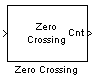# Zero Crossing

Count number of times signal crosses zero in single time step

## Library

Signal Operations

`dspsigops`

•## Description

The Zero Crossing block concludes that a signal in a given channel has passed through zero if it meets any of the following criteria, where xi is the current signal value, xi-1 is the previous signal value, and so on:

• xi < 0 and xi-1 > 0

• xi > 0 and xi-1 < 0

• For some positive integer L, xi < 0, xi-l = 0, and xi-L-1 > 0, where $0\le l\le L$.

• For some positive integer L, xi > 0, xi-l = 0, and xi-L-1 < 0, where $0\le l\le L$.

For the first input value, xi-1 and xi-2 are zero. The block outputs the number of times the signal crosses zero in a single time step at the Cnt port.

The input to this block must be a real-valued fixed-point or floating-point signal. If you set the Input processing parameter to ```Elements as channels (sample based)```, the block treats each element of the input as a time-varying channel. If you set the Input processing parameter to `Columns as channels (frame based)`, the block treats each column of the input as an independent channel.

## Examples

The following example, ex_zero_crossing illustrates the behavior of the Zero Crossing block.

To run the model for one time step, the Stop time is set to `0`.

1. Run the model.

Because the signal passes through zero once during the first time step, the Zero Crossing block finds one zero crossing. The number of detected zero crossings in the first time step is shown in the Display block in the following figure.2. To run the model for two time steps, change the simulation Stop time to `1`. To do so, select Modeling tab and click . In the Solver pane, set Stop time to `1`.

3. Run the model.

The Zero Crossing block remembers that the last value of the last frame was 3. Therefore, the signal passes through zero twice during the second time step. It passes through zero while going from 3 to -3, and it passes through zero again while going from -3 to 3. The Zero Crossing block finds two zero crossings in the second time step as shown in the Display block in the following figure.## Parameters

Input processing

Specify how the block should process the input. You can set this parameter to one of the following options:

• `Columns as channels (frame based)` (default) — When you select this option, the block treats each column of the input as a separate channel.

• `Elements as channels (sample based)` — When you select this option, the block treats each element of the input as a separate channel.

## Supported Data Types

PortSupported Data Types

Input

• Double-precision floating-point

• Single-precision floating-point

• Fixed point (signed and unsigned)

• 8-, 16-, and 32-bit signed integers

• 8-, 16-, and 32-bit unsigned integers

Cnt

• 32-bit unsigned integers